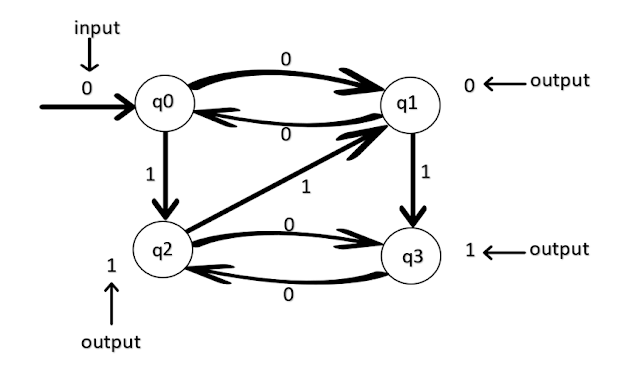# Moore and Mealy Machine | Theory Of Computation (TOC)## Theory Of Computation | (TOC)Moore Machine

Moore machine is an FSM whose outputs depend on only the present state. A Moore machine
can be described by a 6 tuples M = (Q, Σ, Δ, 𝛿, λ, q0)
Where,
Q = ﬁnite set of states.
Σ= finite set of symbols called the input alphabet.
Δ= finite set of symbols called the output alphabet.
𝛿= input transition function where 𝛿: Q✕Σ→Q
λ= output transition function where  λQΔ
q0= initial state from Where any input is processed (q0∈Q).

#### For Example:

A transition table for Moore Machine is like:

Transition Table.

Transition Diagram:

#### OUTPUT From The Machine 000100

In Mealy Machine, if input length = n, then output length = n+1.

## Theory Of Computation | Mealy Machine

A Mealy Machine is an FSM whose output depends on the present state as well as the present input. In this model, all the deﬁnition is same as Moore machine except the output mapping
function λ.
It can be described by 6 tuples M = (Q, Σ, Δ, 𝛿, λ, q0)
Where,
Q = ﬁnite set of states.
Σ= finite set of symbols called the input alphabet.
Δ= finite set of symbols called the output alphabet.
𝛿= input transition function where 𝛿Q✕Σ→Q
λ= output transition function where  λQΣΔ
q0= initial state from where any input is processed (q0∈Q).

Transition Table:

Transition Diagram: